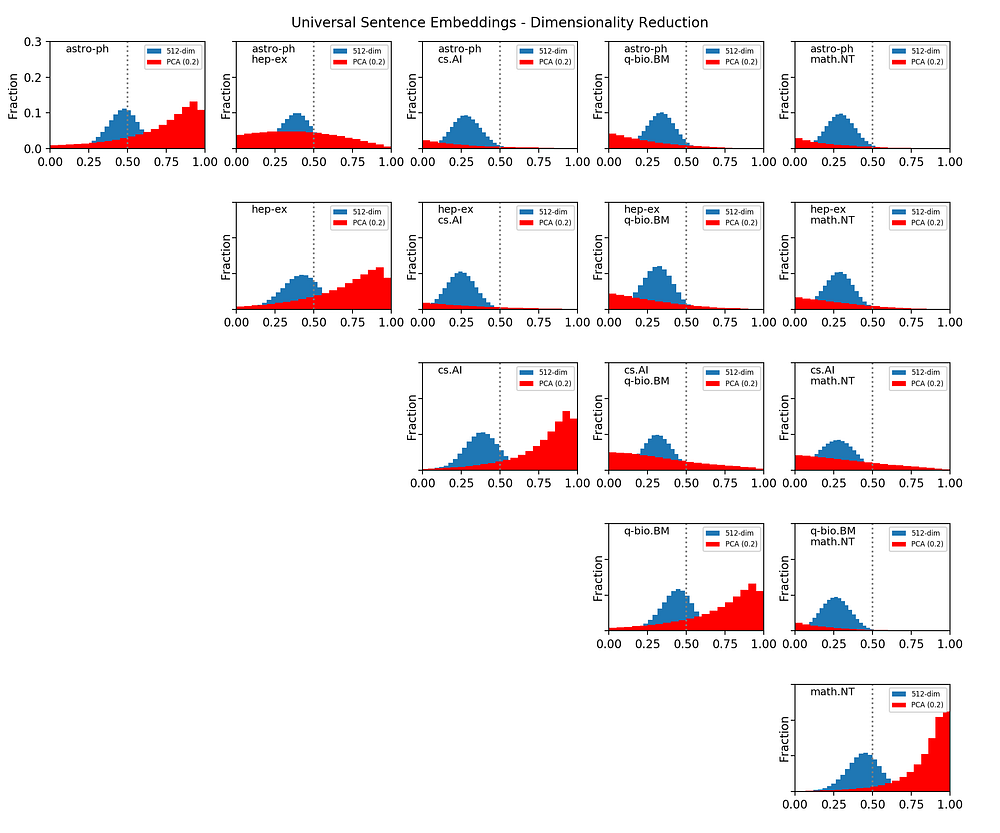# Classifying Scientific Papers with Universal Sentence Embeddings

Source: Deep Learning on Medium## Text Embeddings

While it is quite straightforward to encode an image in terms of numbers that can be manipulated by a computer (i.e. by representing its pixels as a matrix), the same can not be said of a text. In fact, different possibilities exist, and the best depends mostly on the task itself. The most basic method consists of creating a dictionary, i.e. an indexed collection of all the words that appear in the corpus. A given text is then represented by a vector, where each element is the index of the corresponding word.

`dictionary = ["apple", "banana", "zucchini"]"banana banana apple" -> [1, 1, 0]"zucchini apple apple" -> [2, 1, 1]`

The problem with this approach is that the length of the vector is variable (in fact equivalent to the number of words in the text), which is not a desirable feature for numerical processing. Another common choice is to represent the text as a matrix, where each row represents a word. In turn, each word is represented by a fixed-length binary vector where all the elements are zero except a single one that uniquely points to the corresponding word in the dictionary, for example:

`[1,0,0] -> "apple"[0,1,0] -> "banana"[0,0,1] -> "zucchini"`

This method, called one-hot vector, is not particularly suited either because it requires lots of memory, that is basically wasted as most of the elements are zero. The common solution to this problem is to use a sparse-matrix instead.

In recent years, people have realized that an even better solution is to represent each word as dense vectors of fixed length, for example:

`[0.1, -0.1] -> "apple"[0.3, 0.1] -> "banana"[-0.2, 0.1] -> "zucchini"`

The most obvious advantage is that fewer dimensions are needed to encode each word. The dictionary is basically turned into a lookup table of word vectors. The real deal, however, is the ability to “attach” semantic meaning to each dimension of the embedding space. The most glorious example is the following: given four vectors representing king, queen, male, female, one finds that:

`king - male + female = queen`

or similarly:

`france - paris + rome = italy`

How this is achieved goes beyond the scope of this post, but suffice to say that a neural network (word2vec or skip-gram model) is trained on a corpus to predict the most probable context, i.e. the word that follows a given one, or the two words are more likely to be found before and after a given one.

In very recent times, this and other similar tricks have been deployed to train huge networks such as BERT and GPT-2 by crawling millions of web pages (including a full dump of Wikipedia). In this, language-processing networks are doing the same as computer vision ones: their training would be impossible without having access to a massive database of examples, which in turn became possible only with the advent of the Internet.

Finally, and most importantly for our aim, a similar network has been trained by considering not just individual words, but sentences and short paragraphs. We’ll deploy the embedding vectors created by a pre-trained network called Universal Sentence Encoder (USE) to represent documents numerically, and apply some simple classification techniques.